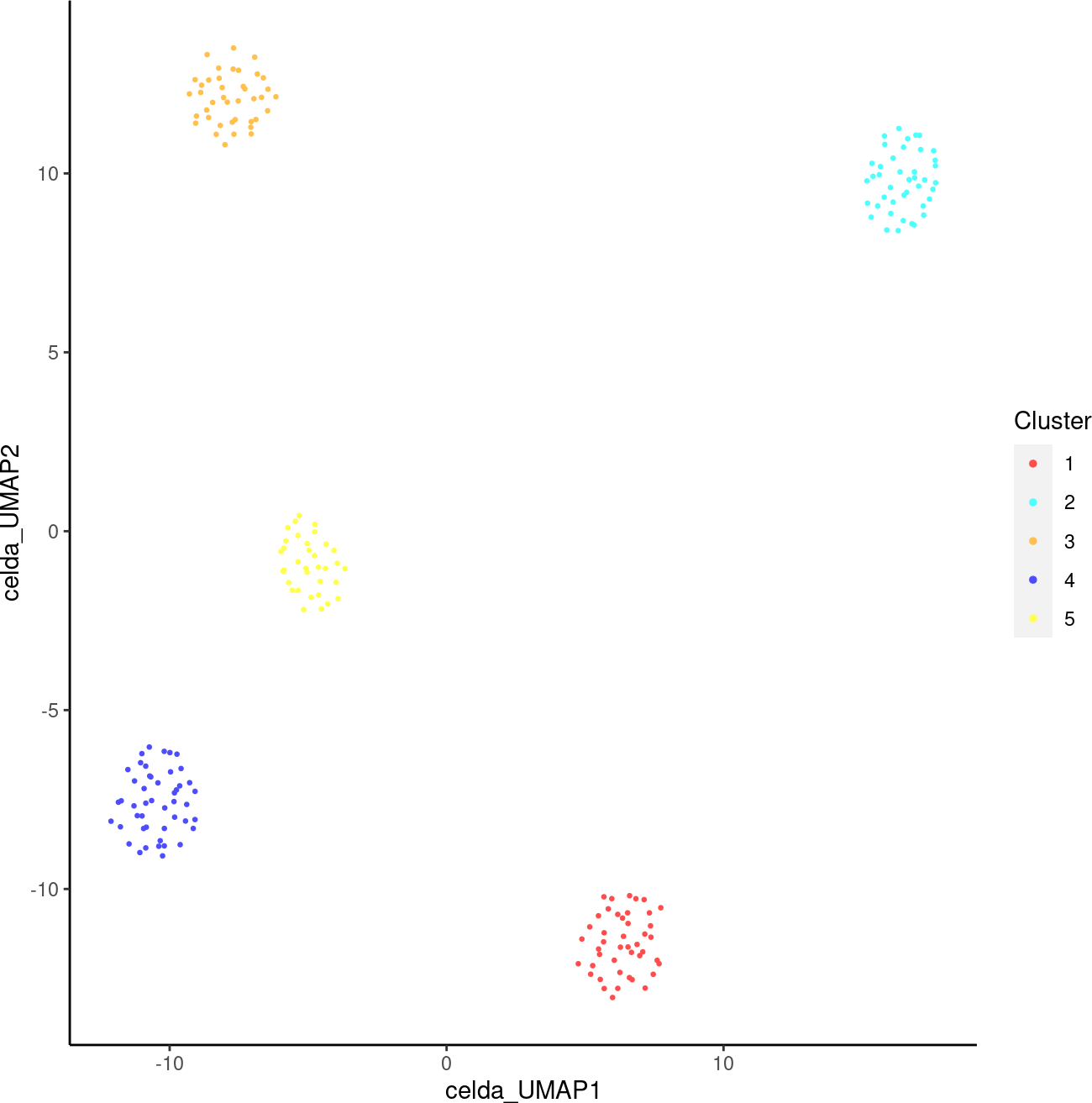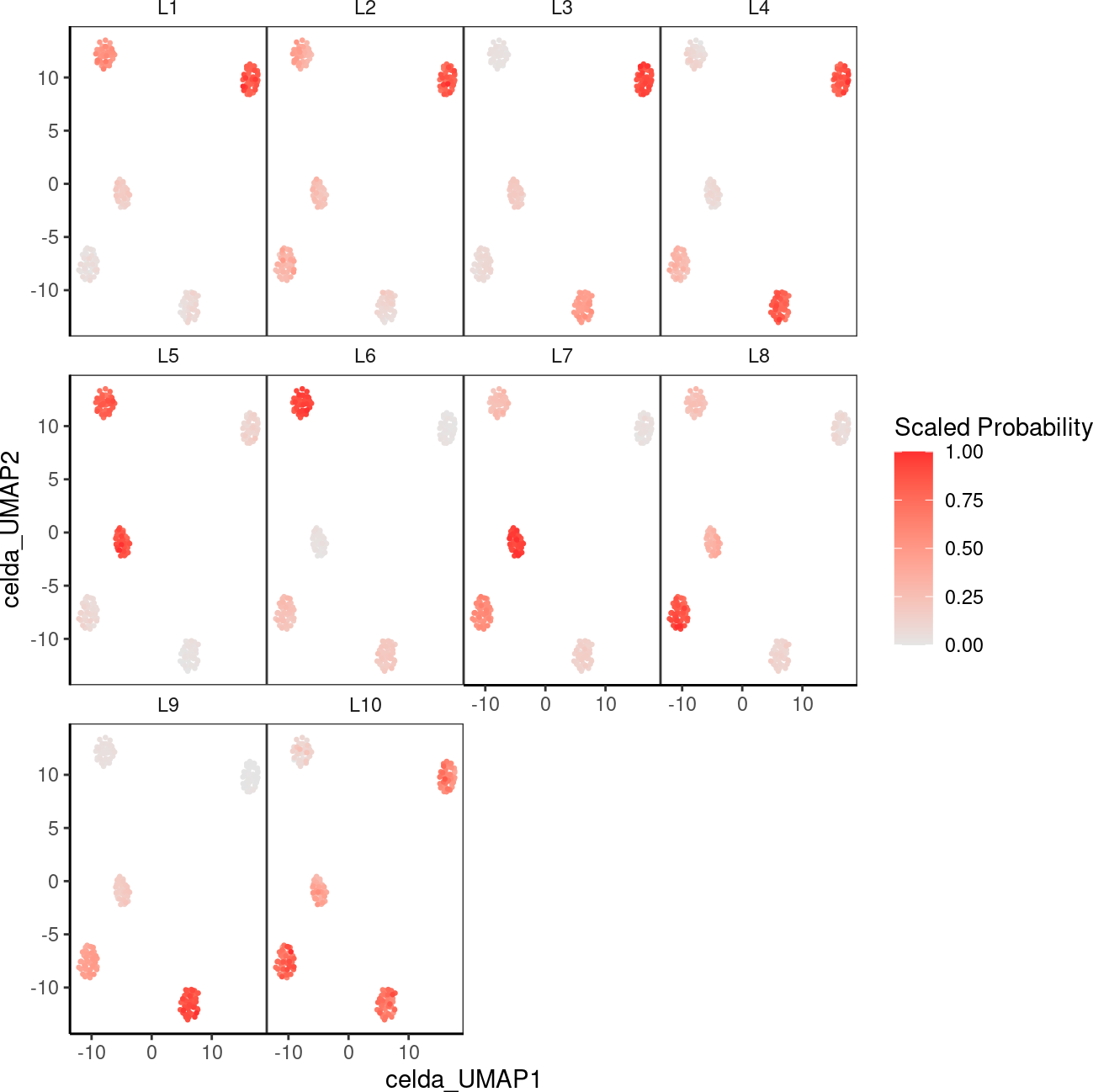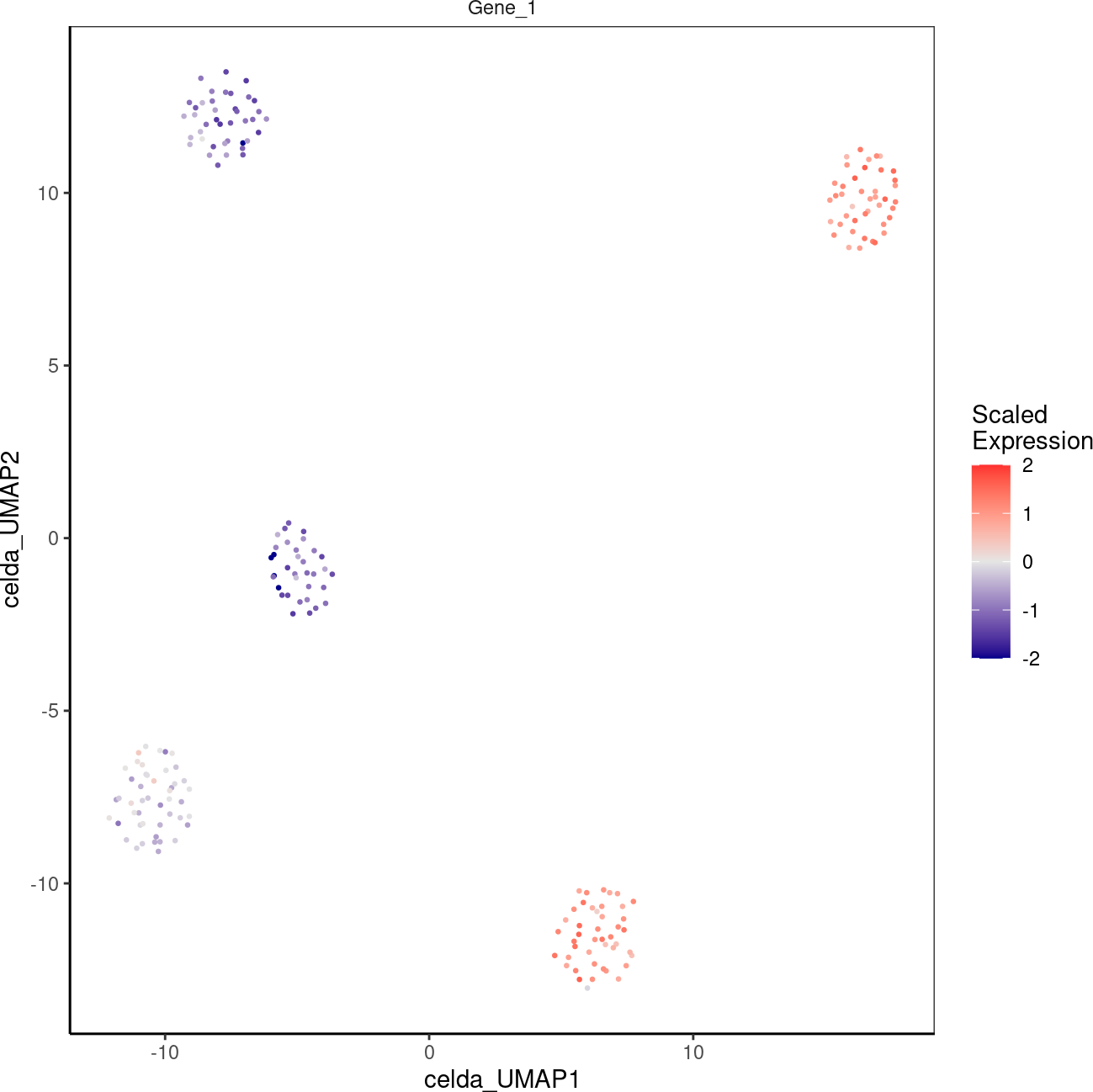# 1 Introduction

CEllular Latent Dirichlet Allocation (celda) is a collection of Bayesian hierarchical models to perform feature and cell bi-clustering for count data generated by single-cell platforms. This algorithm is an extension of the Latent Dirichlet Allocation (LDA) topic modeling framework that has been popular in text mining applications and has shown good performance with sparse data. celda simultaneously clusters features (i.e. gene expression) into modules based on co-expression patterns across cells and cells into subpopulations based on the probabilities of the feature modules within each cell.

Starting from Bioconductor release 3.12 (`celda` version 1.6.0), `celda` makes use of SingleCellExperiment (SCE) objects for storing data and results. In this vignette we will demonstrate how to use celda to perform cell and feature clustering with a simple, small simulated dataset. This vignette does not include upstream importing of data, quality control, or filtering. To see a more complete analysis of larger real-world datasets, visit camplab.net/celda for additional vignettes.

# 2 Installation

celda can be installed from Bioconductor:

``````if (!requireNamespace("BiocManager", quietly = TRUE)) {
install.packages("BiocManager")
}
BiocManager::install("celda")``````

To load the package, type the following:

``library(celda)``

A complete list of help files are accessible using the help command with the `package` option.

``help(package = celda)``

To see the latest updates and releases or to post a bug, see our GitHub page at https://github.com/campbio/celda. To ask questions about running celda, post a thread on Bioconductor support site at https://support.bioconductor.org/.

# 3 Generation of a simulated single cell dataset

celda will take a matrix of counts where each row is a feature, each column is a cell, and each entry in the matrix is the number of counts of each feature in each cell. To illustrate the utility of celda, we will apply it to a simulated dataset.

In the function `simulateCells`, the K parameter designates the number of cell clusters, the L parameter determines the number of feature modules, the S parameter determines the number of samples in the simulated dataset, the G parameter determines the number of features to be simulated, and CRange specifies the lower and upper bounds of the number of cells to be generated in each sample.

To simulate a dataset of 5 samples with 5 cell populations, 10 feature modules, 200 features, and between 30 to 50 cells per sample using `celda_CG` model:

``````simsce <- simulateCells("celda_CG",
S = 5, K = 5, L = 10, G = 200, CRange = c(30, 50))``````

The `counts` assay slot in `simsce` contains the counts matrix. The dimensions of counts matrix:

``````library(SingleCellExperiment)
dim(counts(simsce))``````
``##  200 207``

Columns `celda_sample_label` and `celda_cell_cluster` in `colData(simsce)` contain sample labels and celda cell population cluster labels. Here are the numbers of cells in each subpopulation and in each sample:

``table(colData(simsce)\$celda_cell_cluster)``
``````##
##  1  2  3  4  5
## 42 44 40 47 34``````
``table(colData(simsce)\$celda_sample_label)``
``````##
## Sample_1 Sample_2 Sample_3 Sample_4 Sample_5
##       43       48       45       40       31``````

Column `celda_feature_module` in `rowData(simsce)` contains feature module labels. Here is the number of features in each feature module:

``table(rowData(simsce)\$celda_feature_module)``
``````##
##  1  2  3  4  5  6  7  8  9 10
## 23 39 17 15 21 22 19 12  4 28``````

# 4 Feature selection

A simple heuristic feature selection is performed to reduce the size of features used for clustering. To speed up the process, only features with at least 3 counts in at least 3 cells are included in downstream clustering for this data. A subset `SingleCellExperiment` object with filtered features is stored in `altExp(simsce, "featureSubset")` slot by default.

``simsce <- selectFeatures(simsce)``

If the number of features is still too large, then a smaller subset of features can be obtained by selecting the top number of most variable genes. For an example code, see the PBMC3K tutorial in the online celda documentation.

# 5 Performing bi-clustering with celda

There are currently three models within celda package: `celda_C` will cluster cells, `celda_G` will cluster features, and `celda_CG` will simultaneously cluster cells and features. Within the functions the `K` parameter will be the number of cell populations to be estimated, while the `L` parameter will be the number of feature modules to be estimated in the output model.

``sce <- celda_CG(x = simsce, K = 5, L = 10, verbose = FALSE, nchains = 1)``

Here is a comparison between the true cluster labels and the estimated cluster labels.

``table(celdaClusters(sce), celdaClusters(simsce))``
``````##
##      1  2  3  4  5
##   1  0 44  0  0  0
##   2 42  0  0  0  0
##   3  0  0 40  0  0
##   4  0  0  0 47  0
##   5  0  0  0  0 34``````
``table(celdaModules(sce), celdaModules(simsce))``
``````##
##       1  2  3  4  5  6  7  8  9 10
##   1   0 32  0  0  0  0  0  0  0  0
##   2  19  0  0  0  0  0  0  0  0  0
##   3   0  0 15  0  0  0  0  0  0  0
##   4   0  0  0 13  0  0  0  0  0  0
##   5   0  0  0  0 21  0  0  0  0  0
##   6   0  0  0  0  0 19  0  0  0  0
##   7   0  0  0  0  0  0  0 12  0  0
##   8   0  0  0  0  0  0 17  0  0  0
##   9   0  0  0  0  0  0  0  0  3  0
##   10  0  0  0  0  0  0  0  0  0 20``````

# 6 Visualization

## 6.1 Plotting cell populations on 2D-embeddings

celda contains its own wrapper function for tSNE and UMAP called `celdaTsne` and `celdaUmap`, respectively. Both of these functions can be used to embed cells into 2-dimensions. The output can be used in the downstream plotting functions `plotDimReduceCluster`, `plotDimReduceModule`, and `plotDimReduceFeature` to show cell population clusters, module probabilities, and expression of individual features, respectively.

``sce <- celdaUmap(sce)``
``plotDimReduceCluster(x = sce, reducedDimName = "celda_UMAP")````plotDimReduceModule(x = sce, reducedDimName = "celda_UMAP", rescale = TRUE)````````plotDimReduceFeature(x = sce, reducedDimName = "celda_UMAP",
normalize = TRUE, features = "Gene_1")``````## 6.2 Creating an expression heatmap

The clustering results can be viewed with a heatmap of the normalized counts using the function `celdaHeatmap`. The top `nfeatures` in each module will be selected according to the factorized module probability matrix.

``plot(celdaHeatmap(sce = sce, nfeatures = 10))``